Arquímedes: Area of an ellipse (II)

Knowing the result, Archimedes considered a secondary circle with the same area than the ellipse.The radius of this circle is:

Archimedes wanted to probe that the area of the ellipse is equal to the area of this secondary circle

Archimedes considered an auxiliary polygon similar to P', the polygon inscribed in the auxiliary circle C'.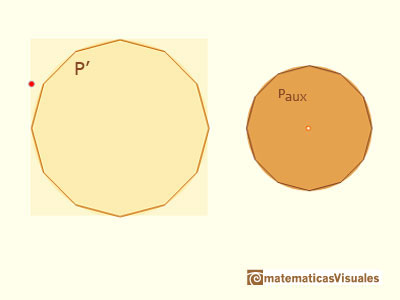The relation between the areas of these two similar polygons is:

Archimedes estarted his double 'reductio ad absurdum'.If posible, let the secundary circle greater than the ellipse."We can then inscribe in the secundary circle an equilateral polygon of 4n sides such that its area is greater than that of the ellipse. [cf. On the Sphere and Cylinder, I. 6.]" (Archimedes)

ThenThen we can consider a similar polygon in the auxiliary circle and the corresponding polygon in the ellipse.

"Supose that P' denotes the area of the polygon inscribed in the auxiliary circle, and P that of the polygon inscribed in the ellipse." (Archimedes)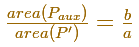Then

"But this is imposible, because the later polygon is by hypothesis greater than the ellipse, and a fortiory greater than P.Hence the secondary circle is not greater than the ellipse." (Archimedes)If posible, let the secundary circle be less than the ellipse.In this case we inscribe in the ellipse a polygon P with 4n equal sides such that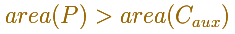Archimedes consider polygon P' inscribed to the auxiliary circle and a similar polygon inscribed in the secondary circle.

As before

which is imposibleThis completes the double reductio ad absurdum proof.

"Hence the secondary circle, being neither greater nor less than the ellipse, si equal to it; and the required result follows."(Archimedes)

"In esence, Archimedes has simply given a rigorous exhaustion proof of the intuitively evident fact that the area of the ellipse is b/a times the area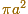of its auxiliary circle, corresponding to the observation that the circle is transformed into the ellipse by shrinking its vertical dimension by the factor b/a" (C. H. Edwards)

REFERENCES

C.H. Edwards - The Historical Development of the Calculus (pag. 40-42) - Springer-Verlag New York Inc.
Archimedes - On Conoids and Spheroids -- The Works of Archimedes edited by T.L. Heath - Dover Publications, Inc.Plane developments of cones cut by an oblique plane. The section is an ellipse.Transforming a circle we can get an ellipse (as Archimedes did to calculate its area). From the equation of a circle we can deduce the equation of an ellipse.Every ellipse has two foci and if we add the distance between a point on the ellipse and these two foci we get a constant.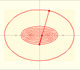An Ellipsograph is a mechanical device used for drawing ellipses.If a straight-line segment is moved in such a way that its extremities travel on two mutually perpendicular straight lines then the midpoint traces out a circle; every other point of the line traces out an ellipse.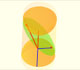The section of a cylinder by a plane cutting its axis at a single point is an ellipse. A beautiful demonstration uses Dandelin Spheres.Durer was the first who published in german a method to draw ellipses as cone sections.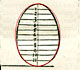Durer made a mistake when he explanined how to draw ellipses. We can prove, using only basic properties, that the ellipse has not an egg shape .The Astroid is the envelope of a segment of constant length moving with its ends upon two perpendicular lines. It is also the envelope of a family of ellipses, the sum of whose axes is constant.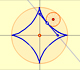The Astroid is a particular case of a family of curves called hypocycloids.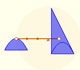Archimedes show us in 'The Method' how to use the lever law to discover the area of a parabolic segment.Kepler used an intuitive infinitesimal approach to calculate the area of a circle.He studied transformations of images, for example, faces.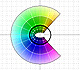The Complex Cosine Function extends the Real Cosine Function to the complex plane. It is a periodic function that shares several properties with his real ancestor.